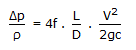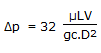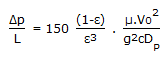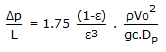# Chemical Engineering - Fluid Mechanics

36.

Pick out the Hagen-Poiseulle's equation.

 A.B.C.D.Explanation:

No answer description available for this question. Let us discuss.

37.

In case of isentropic flow, the speed of sound in an ideal gas is proportional to (where M = molecular weight of the gas)

 A. 1/M B. M C. 1/M D. M

Explanation:

No answer description available for this question. Let us discuss.

38.

Boundary layer separation is caused by the

 A. reduction of pressure to vapour pressure. B. boundary layer thickness reducing to zero. C. adverse pressure gradient. D. reduction of pressure gradient to zero.

Explanation:

No answer description available for this question. Let us discuss.

39.

With increase in temperature, the vapor pressure of liquids

 A. increases B. increases linearly C. decreases D. remains constant

Explanation:

No answer description available for this question. Let us discuss.

40.

A mercury (specific gravity = 13.6) manometer connected across an orificemeter fitted in a pipe shows a manometer reading of 2 cms. If the manometer liquid is changed to carbon tetrachloride (specific gravity = 1.6), then for the same flow rate of water the new manometer reading will be __________ cms.

 A. 17 B. 42 C. 84 D. 1.8